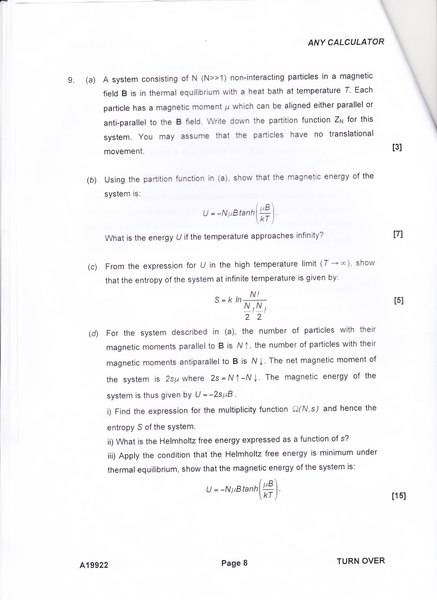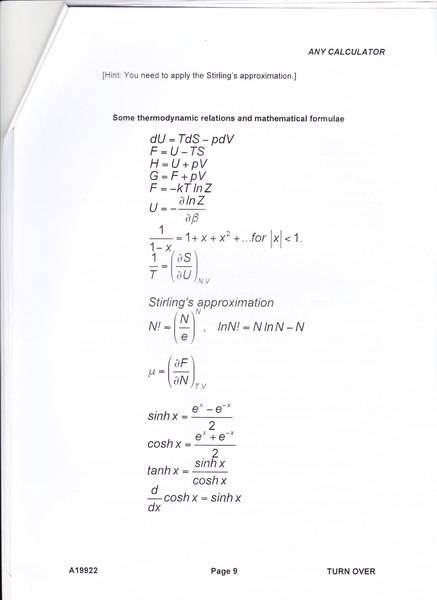# Statistical physics question - particles in a magnetic field

• subzero0137

## Homework Statement

[/B]
I'm stuck on part (b) and (c) of the following question## Homework Equations## The Attempt at a Solution

The partition function was ##Z_N = 2 cosh(μBβ)## where ##β = \frac {1}{kT}##. From there I used ##U = - \frac {∂}{∂β} ln (Z_n)## to get ##U = -NμB tanh( \frac {μB}{kT})##.

But I'm stuck on the part where it says "What is the energy U if the temperature approaches infinity?" Wouldn't the energy U be zero if the temperature approaches infinity? But then what does it mean in part (c) where it's assuming we have an EXPRESSION for U in the T→∞ limit? What is that expression? And how do I go about determining the entropy S of the system at infinite temperature?

#### Attachments

I recommend you consider the case of large ## T ## and do a Taylor expansion of the component parts of ## \tanh{x} ## for large ##T ##, which is small ##x ##. ## \\ ## See https://en.wikipedia.org/wiki/Partition_function_(statistical_mechanics) for how to compute the entropy.

Okay, I got tanh(x) ≈ x from the Taylor expansion, so I managed to obtain the expression ##U = - \frac {N (μB)^2}{kT}## for the T→∞ limit. Is this correct?

I'm still confused about the entropy part. Am I supposed to calculate the helmholtz energy first?

I'm still confused about the entropy part. Am I supposed to calculate the helmholtz energy first?
Yes. I believe so. I'm no expert at Statistical Physics, but I've had a couple of courses in it including a graduate level class or two. Computing the Helmholtz Free Energy first is normally how that calculation would proceed. ## \\ ## And I didn't look through your calculation of ## U ## in full detail, but it looks correct. Qualitatively, you can expect an additional fraction ##f \approx \frac{\mu B}{k T} ## of the ## N ## spins to be aligned, rather than a 50-50 distribution of both directions. The energy of each extra one that is aligned is ## u_s=-\mu B ##. (With a 50-50 distribution, the energy ## U=0 ##).

Last edited:
Yes. I believe so. I'm no expert at Statistical Physics, but I've had a couple of courses in it including a graduate level class or two. Computing the Helmholtz Free Energy first is normally how that calculation would proceed. ## \\ ## And I didn't look through your calculation of ## U ## in full detail, but it looks correct. Qualitatively, you can expect an additional fraction ##f \approx \frac{\mu B}{k T} ## of the ## N ## spins to be aligned, rather than a 50-50 distribution of both directions. The energy of each extra one that is aligned is ## u_s=-\mu B ##. (With a 50-50 distribution, the energy ## U=0 ##).

Hmm, I'm still not able to get the expression for entropy at infinite T as given in the question. Here's my attempt so far:

$$S = - \frac {∂A}{∂T} = - \frac {∂}{∂T} (-kT ln(Z_N)$$ where ##Z_N = (2 cosh(\frac {μB}{kT})^N##. When T→∞, cosh(x)→1 so ##Z_N = 2^N##. Therefore $$S = k ln(2^N)$$
But how do I get $$S = k ln \left(\frac {N!}{\frac {N}{2}! \frac{N}{2}!} \right)$$?

Try taking the derivative first, before yot let ## T \rightarrow +\infty ##. Let me try to work it, and see if I can get their result.

Try taking the derivative first, before yot let ## T \rightarrow +\infty ##. Let me try to work it, and see if I can get their result.

I got $$S = -Nk \frac {μB}{kT} tanh (\frac {μB}{kT}) + ln (2cosh(\frac{μB}{kT}))$$. Letting T→∞ gives ##S = -Nk (\frac{μB}{kT})^2 + ln 2##. Something doesn't seem right. I didn't even get to use the expression for U at T→∞ to get this entropy even though the question wants me to use it?

I just got it to work=let me show it to you momentarily...
Starting with ## A=-kT \ln({2^N}) ##, ##S=-\frac{\partial{A}}{\partial{T}}=k \ln{(2^N)} =Nk \ln{2} ##.
Meanwhile they give ##S=k \ln{(\frac{N!}{((N/2)!)^2})} ##. Using Stirlings: ##S=k[N \ln{N}-N-2(\frac{N}{2} \ln{(N/2)}-(N/2))]=k[N \ln{N}-N-(N \ln{N}-N \ln{2})+N]=Nk \ln{2} ##. ## \\ ## I don't know that their answer is exact. Using Stirling's on their answer gives you the exact answer. ## \\ ## In your previous post, you left off the exponent of ## N ## on the second term, along with a ## k ##. ## S=\beta U +k \ln{Z} ##.

Last edited:
•subzero0137
Additional comment on the above. Their answer for the entropy at high temperatures uses the combinations of ## N ## things taken ## \frac{N}{2} ## at a time. This also is logical. ## \\ ## For part d, ## s=N_+-\frac{N}{2} ##, so that ## N_+=s+\frac{N}{2} ##. I think their ## \Omega ## can easily and immediately be written in terms of ## N ## and ## N_+ ##.

Additional comment on the above. Their answer for the entropy at high temperatures uses the combinations of ## N ## things taken ## \frac{N}{2} ## at a time. This also is logical. ## \\ ## For part d, ## s=N_+-\frac{N}{2} ##, so that ## N_+=s+\frac{N}{2} ##. I think their ## \Omega ## can easily and immediately be written in terms of ## N ## and ## N_+ ##.

$$S = k ln Ω(N,s) = k ln \frac {N!}{\left (\frac{N}{2} + s \right)! \left (\frac {N}{2} - s \right)!}$$

Applying Stirling's approximation gives:

$$S = k \left [NlnN - \left (\frac {N}{2} + s \right) ln \left (\frac {N}{2} + s \right) - \left (\frac {N}{2} - s \right) ln \left (\frac {N}{2} - s \right)\right]$$.

For part d)ii) it's asking for the Helmholtz free energy as a function of s. In the attached formula sheet Helmholtz free energyis given by ##F = U - TS##. So I'm guessing in this case U is given as ##U=-2sμB## in the question itself and we just obtained an expression for S, so $$F = -2sμB - Tk\left [NlnN - \left (\frac {N}{2} + s \right) ln \left (\frac {N}{2} + s \right) - \left (\frac {N}{2} - s \right) ln \left (\frac {N}{2} - s \right)\right]$$? If this is correct so far, in part d)iii) it asks us to the apply the condition that Helmholtz free energy is minimum under thermal equilibrium and show that the magnetic energy of the system is given by $$U=-NμB tanh(\frac {μB}{kT})$$, which is the same as the expression I got in part (a) from the partition function. I'm not sure how to apply this minimum condition to get the expression.

•The first part is exactly what I was thinking, and for the last part, I think all you need to do is set ## \frac{dF}{ds}=0 ##. I think if you try that, you likely will get the result they are looking for. That will give you the ## s ## you need, and just plug it back into ## U ##. ## \\ ## And yes, I got it to work.

Last edited:
The first part is exactly what I was thinking, and for the last part, I think all you need to do is set ## \frac{dF}{ds}=0 ##. I think if you try that, you likely will get the result they are looking for. That will give you the ## s ## you need, and just plug it back into ## U ##. ## \\ ## And yes, I got it to work.

I got $$s = \frac {N}{2} \frac {e^{\frac {2μB}{kT} - 1}}{1 + e^{\frac{2μB}{kT}}}$$

Should I plug this in U?

Last edited:
•First multiply numerator and denominator by ## e^{-(\frac{\mu B}{kT})} ##. Then plug it in. (And it is little "s", not capital S). ## \\ ## You basically have it. Very good.## \\ ## ## s=\frac{N}{2} \tanh{(\frac{\mu B}{kT})} ## ## \\ ## ## U=-2s \mu B ##.
•